Скачать презентацию Construction of Global Phase Equilibrium Diagrams Martín Cismondi

• Количество слайдов: 51Construction of Global Phase Equilibrium Diagrams Martín Cismondi Universidad Nacional de Córdoba - CONICET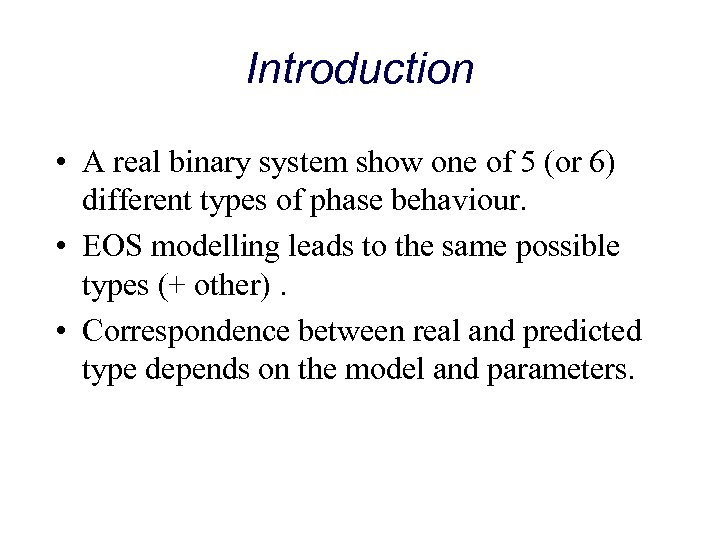Introduction • A real binary system show one of 5 (or 6) different types of phase behaviour. • EOS modelling leads to the same possible types (+ other). • Correspondence between real and predicted type depends on the model and parameters.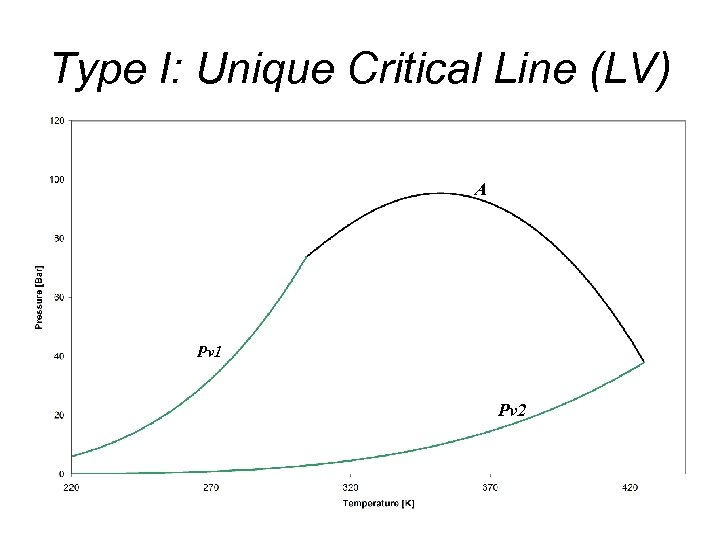Type I: Unique Critical Line (LV) A Pv 1 Pv 2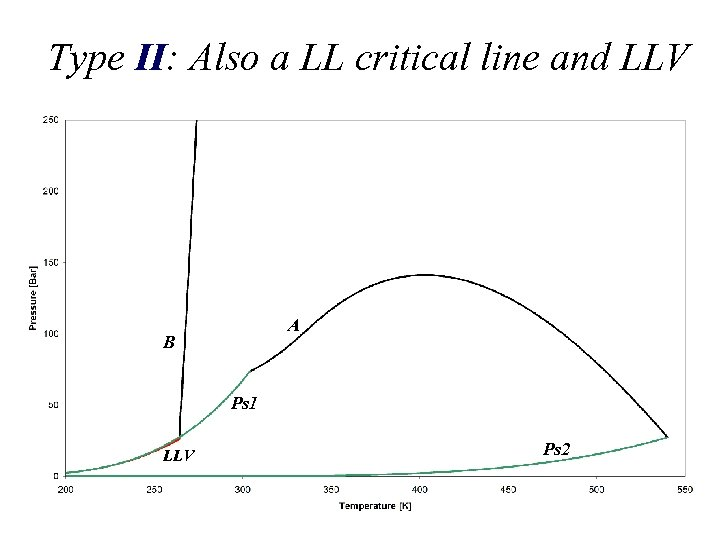Type II: Also a LL critical line and LLV A B Ps 1 LLV Ps 2Type IV: Discontinuity in the LV critical line and second LLV region E UCEP LLV D LCEP E B D LLVType IV: T-x projection E UCEP LCEP B UCEP DType III: “rearrangement” of critical lines C D UCEP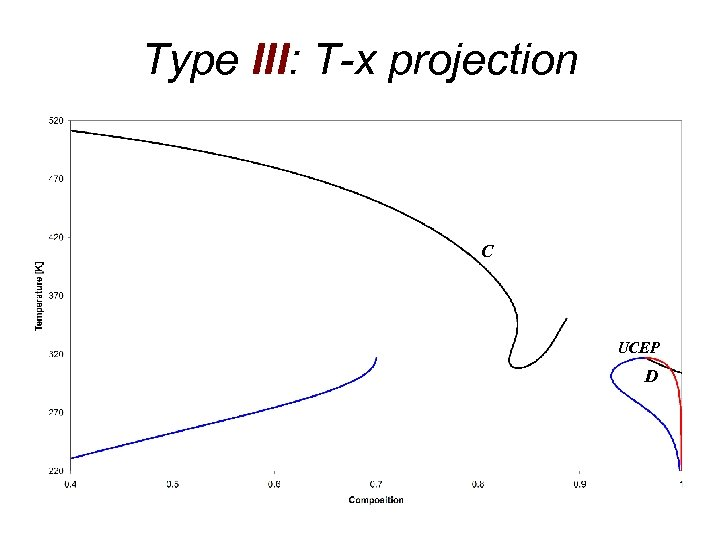Type III: T-x projection C UCEP D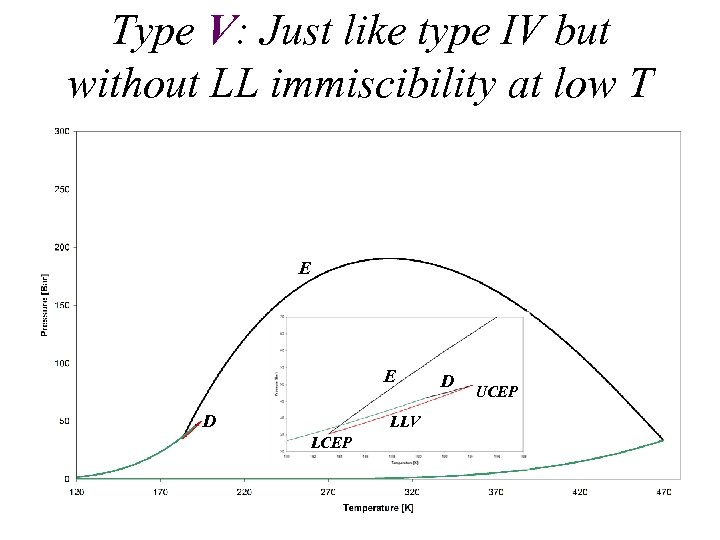Type V: Just like type IV but without LL immiscibility at low T E E D LLV LCEP D UCEP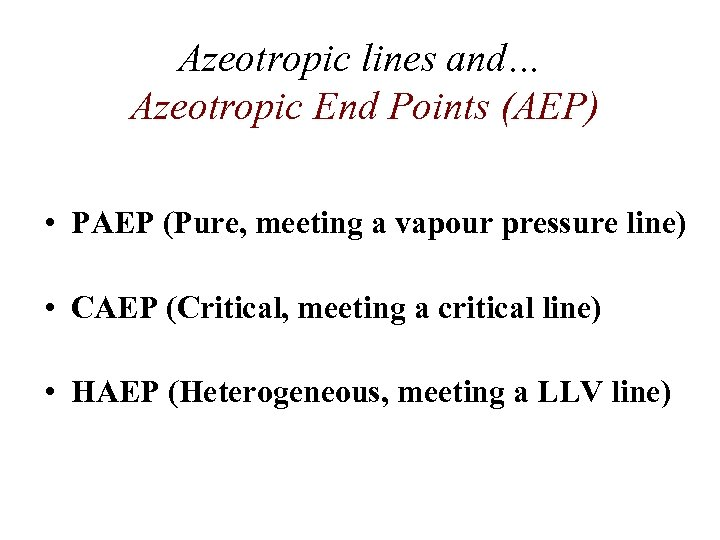Azeotropic lines and… Azeotropic End Points (AEP) • PAEP (Pure, meeting a vapour pressure line) • CAEP (Critical, meeting a critical line) • HAEP (Heterogeneous, meeting a LLV line)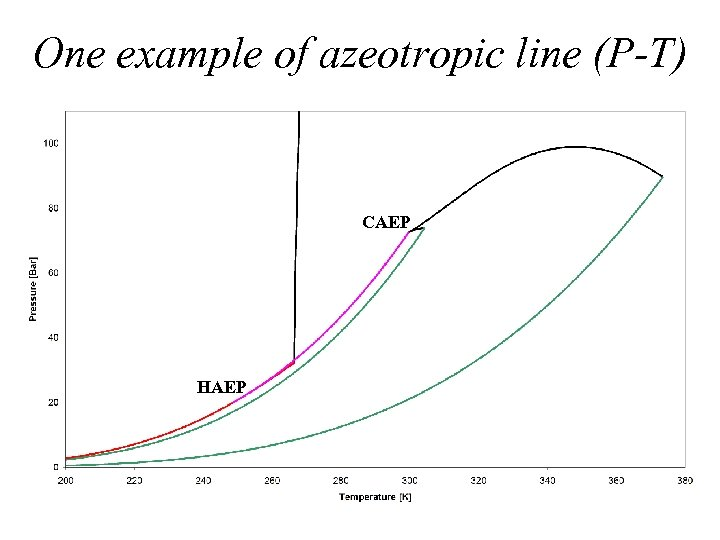One example of azeotropic line (P-T) CAEP HAEP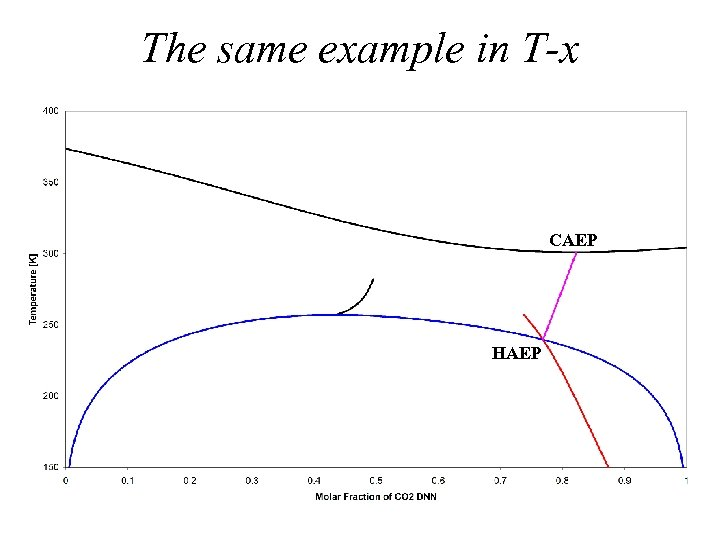The same example in T-x CAEP HAEP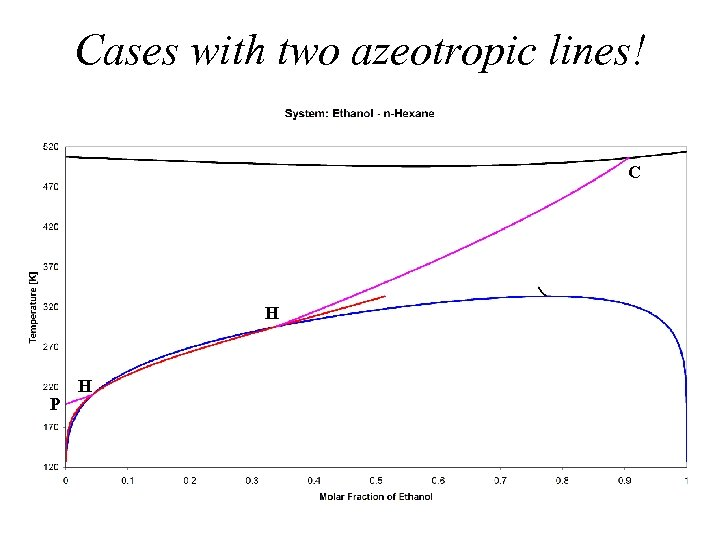Cases with two azeotropic lines! C H P H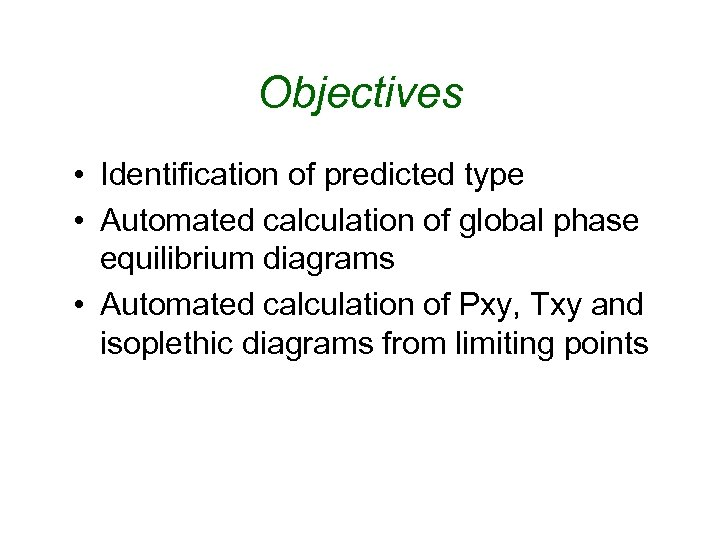Objectives • Identification of predicted type • Automated calculation of global phase equilibrium diagrams • Automated calculation of Pxy, Txy and isoplethic diagrams from limiting points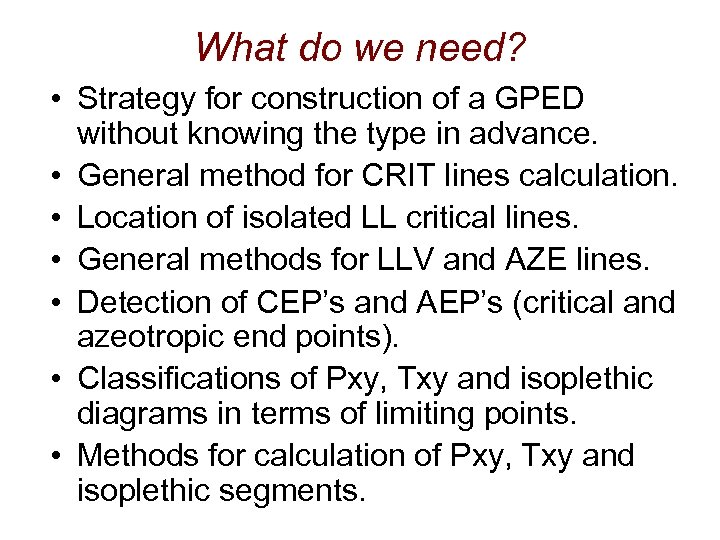What do we need? • Strategy for construction of a GPED without knowing the type in advance. • General method for CRIT lines calculation. • Location of isolated LL critical lines. • General methods for LLV and AZE lines. • Detection of CEP’s and AEP’s (critical and azeotropic end points). • Classifications of Pxy, Txy and isoplethic diagrams in terms of limiting points. • Methods for calculation of Pxy, Txy and isoplethic segments.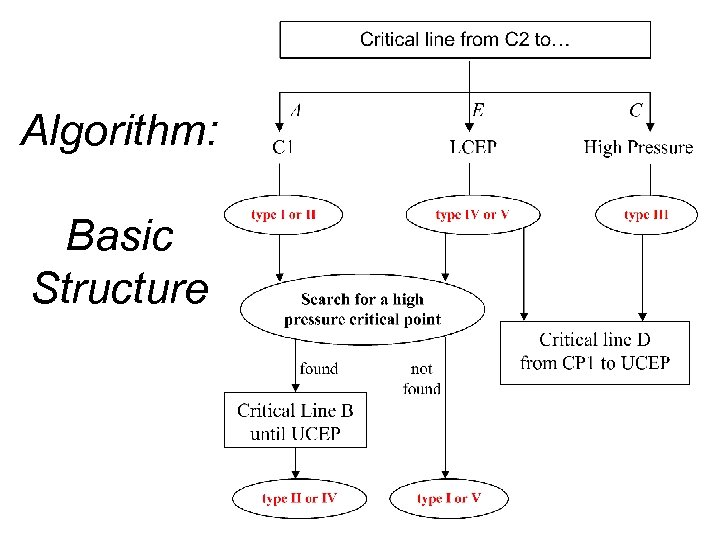Algorithm: Basic Structure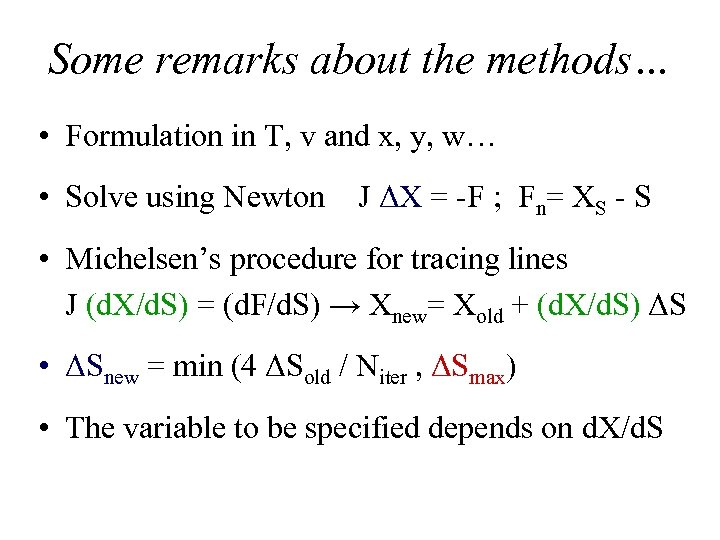Some remarks about the methods… • Formulation in T, v and x, y, w… • Solve using Newton J ΔX = -F ; Fn= XS - S • Michelsen’s procedure for tracing lines J (d. X/d. S) = (d. F/d. S) → Xnew= Xold + (d. X/d. S) ΔS • ΔSnew = min (4 ΔSold / Niter , ΔSmax) • The variable to be specified depends on d. X/d. S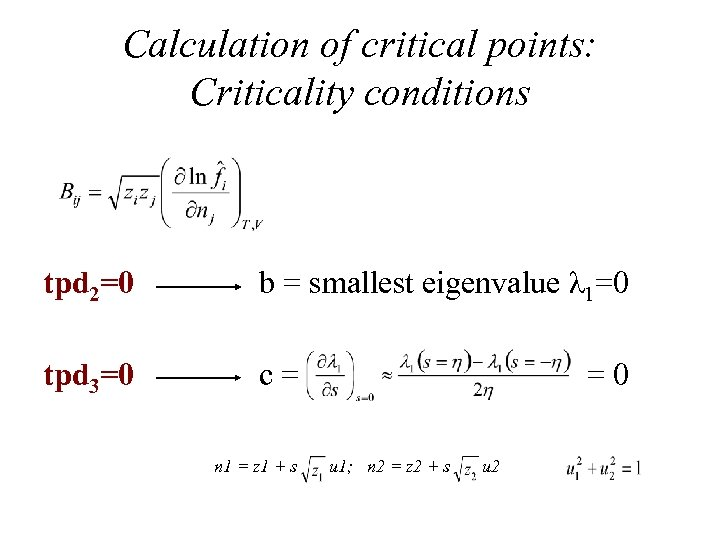Calculation of critical points: Criticality conditions tpd 2=0 b = smallest eigenvalue λ 1=0 tpd 3=0 c = n 1 = z 1 + s = 0 u 1; n 2 = z 2 + s u 2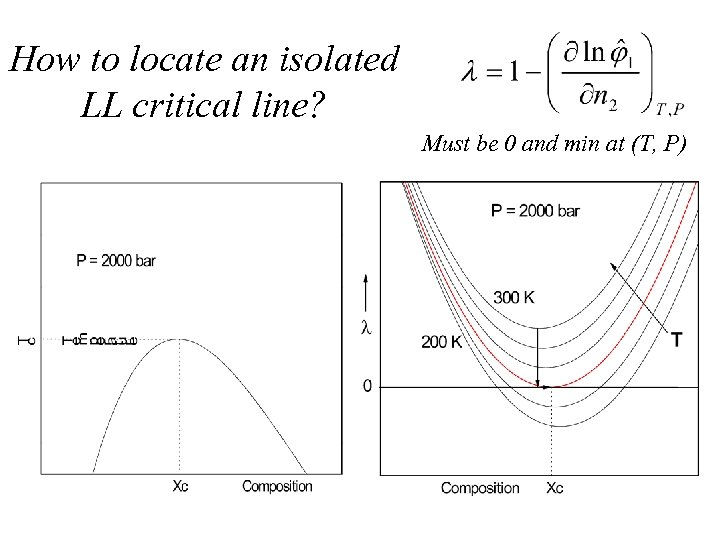How to locate an isolated LL critical line? Must be 0 and min at (T, P)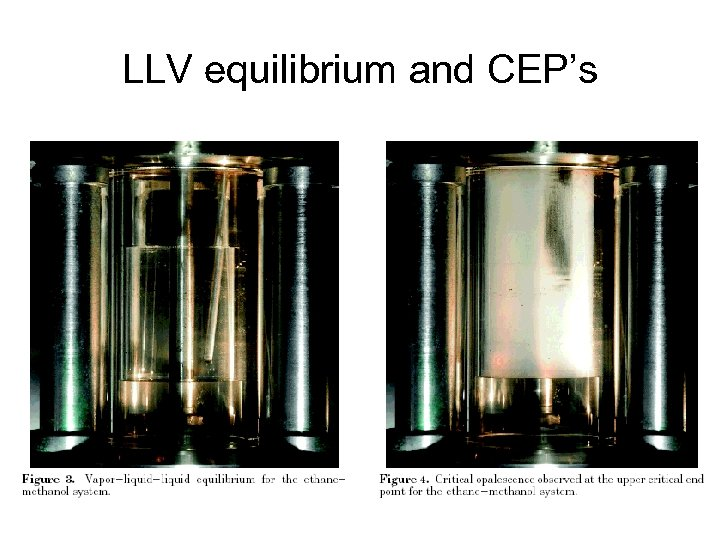LLV equilibrium and CEP’sUse of stability analysis in the search for a Critical End Point (CEP)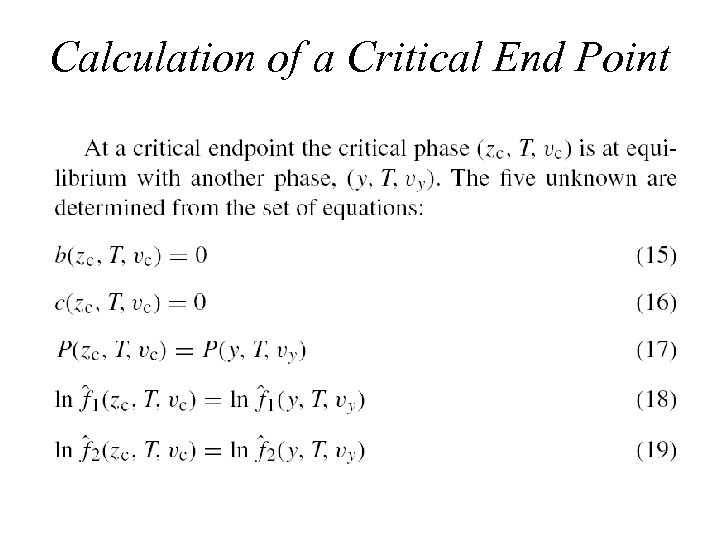Calculation of a Critical End Point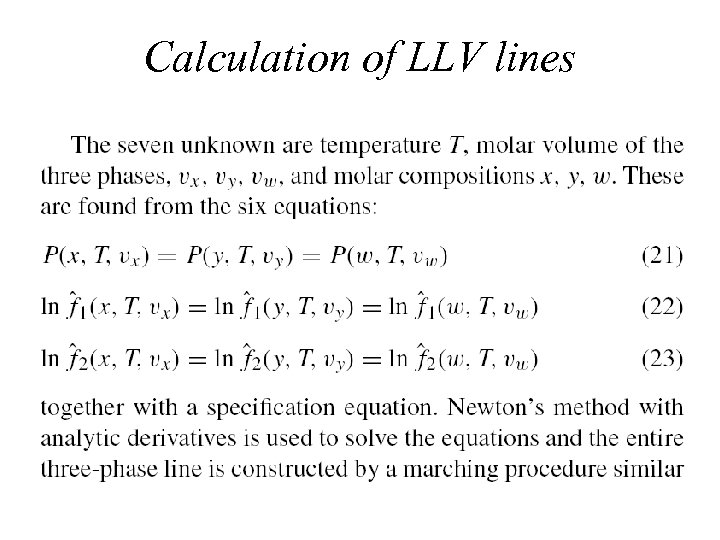Calculation of LLV linesExamples: type II minimum composition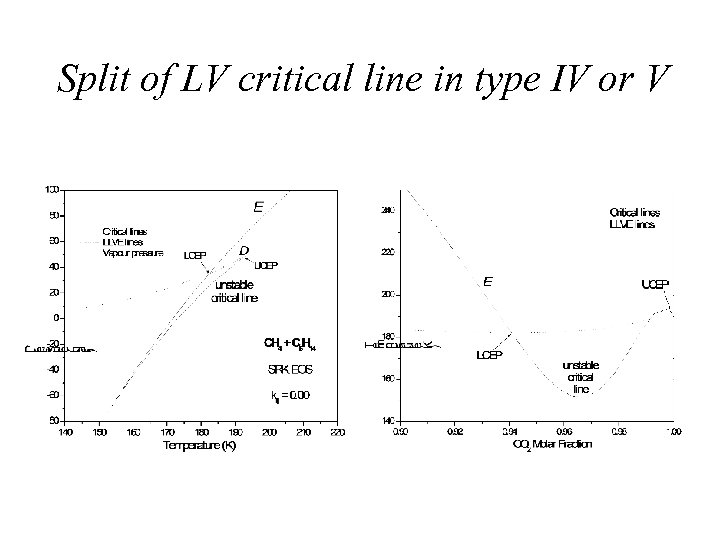Split of LV critical line in type IV or V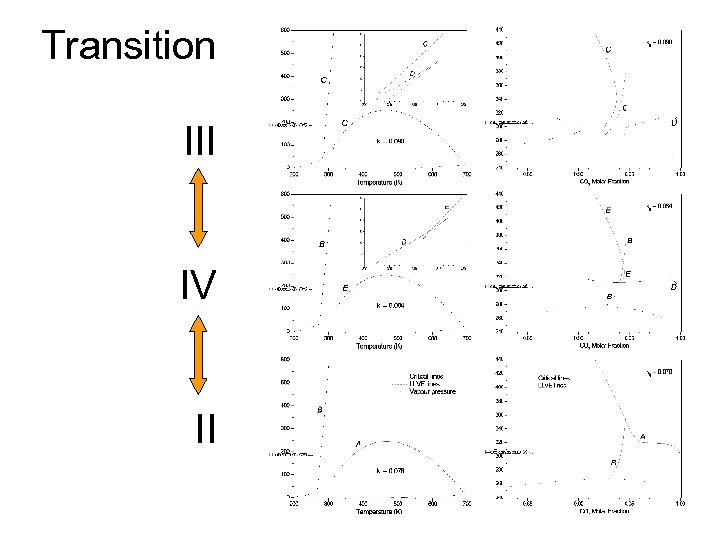Transition III IV II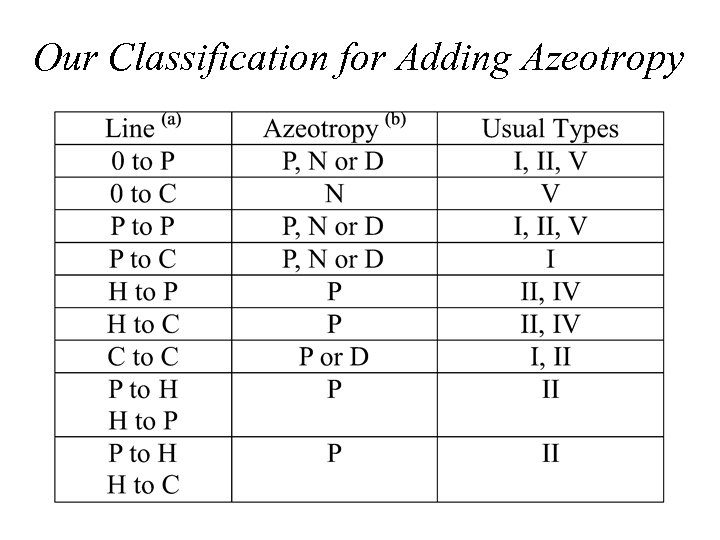Our Classification for Adding Azeotropy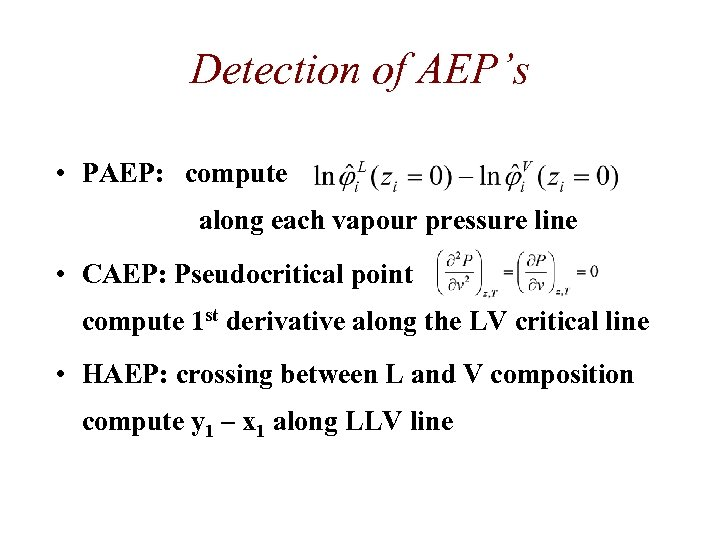Detection of AEP’s • PAEP: compute along each vapour pressure line • CAEP: Pseudocritical point compute 1 st derivative along the LV critical line • HAEP: crossing between L and V composition compute y 1 – x 1 along LLV line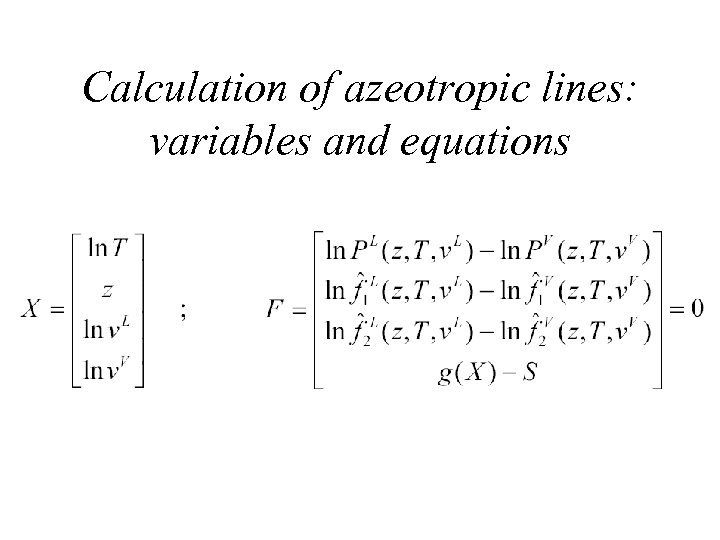Calculation of azeotropic lines: variables and equationsIllustration: Negative Azeotropy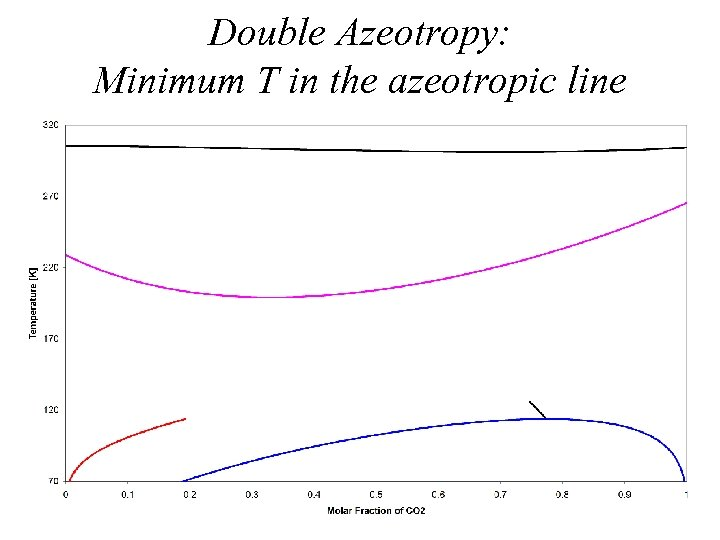Double Azeotropy: Minimum T in the azeotropic line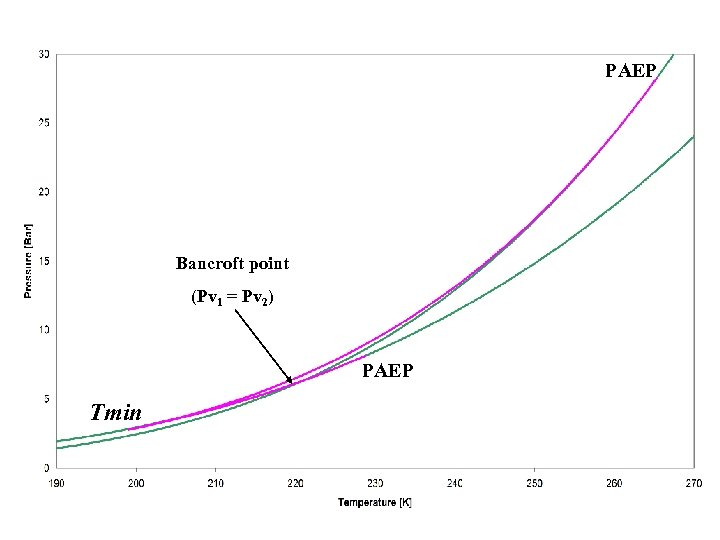PAEP Bancroft point (Pv 1 = Pv 2) PAEP Tmin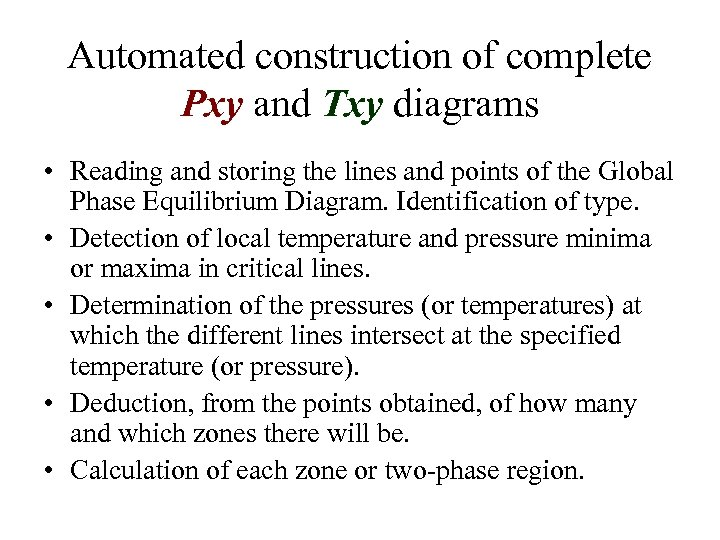Automated construction of complete Pxy and Txy diagrams • Reading and storing the lines and points of the Global Phase Equilibrium Diagram. Identification of type. • Detection of local temperature and pressure minima or maxima in critical lines. • Determination of the pressures (or temperatures) at which the different lines intersect at the specified temperature (or pressure). • Deduction, from the points obtained, of how many and which zones there will be. • Calculation of each zone or two-phase region.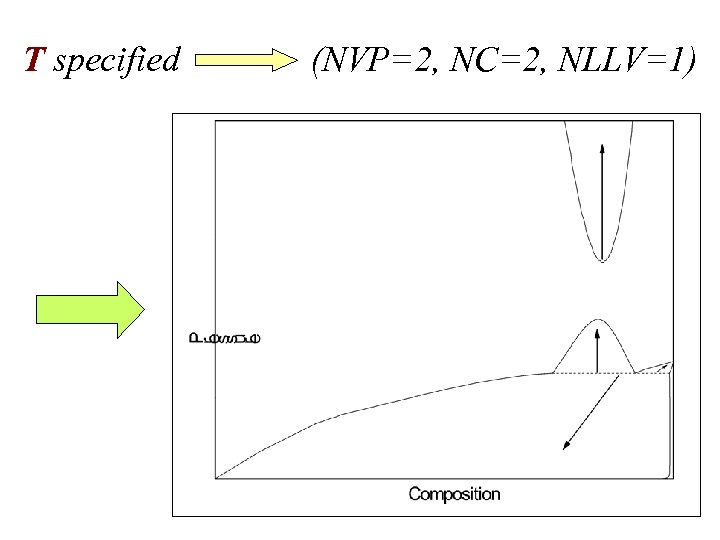T specified (NVP=2, NC=2, NLLV=1)Translating from limiting points to diagrams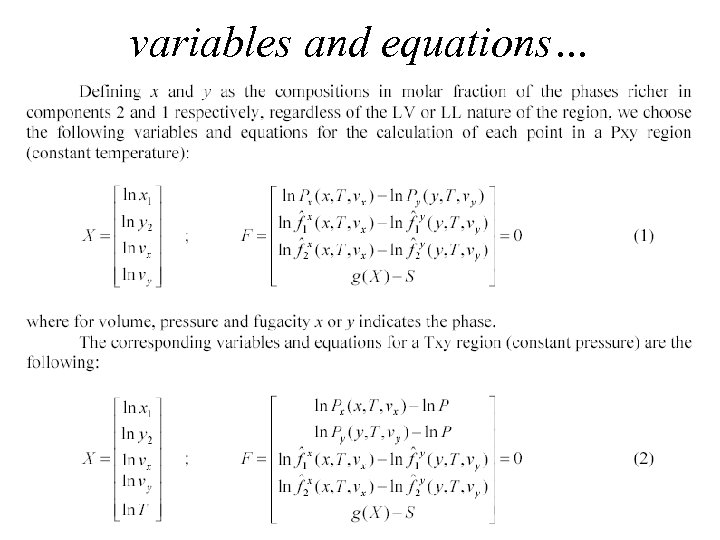variables and equations…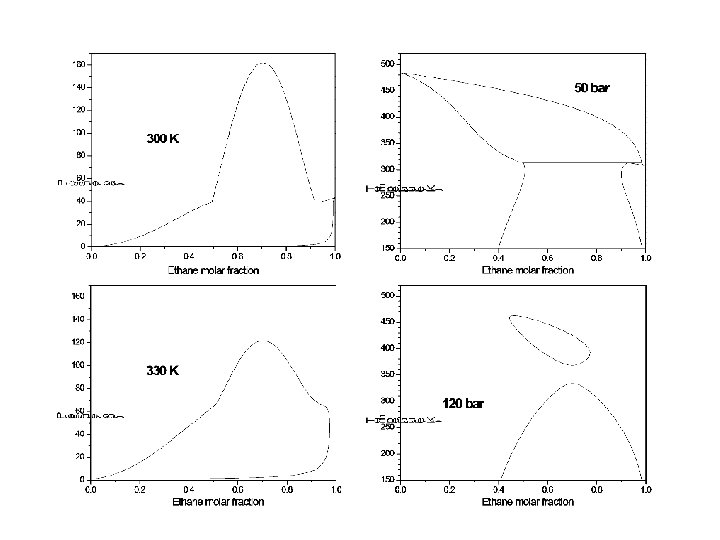Examples: Closed loops in Pxy diagrams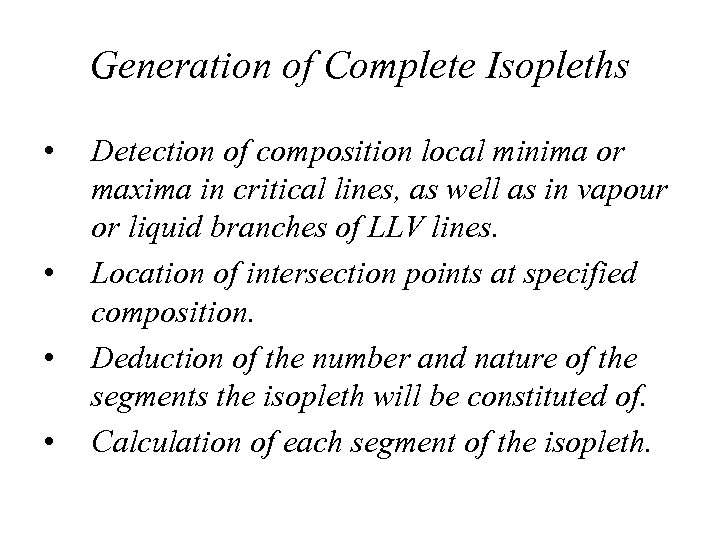Generation of Complete Isopleths • • Detection of composition local minima or maxima in critical lines, as well as in vapour or liquid branches of LLV lines. Location of intersection points at specified composition. Deduction of the number and nature of the segments the isopleth will be constituted of. Calculation of each segment of the isopleth.Location of intersection points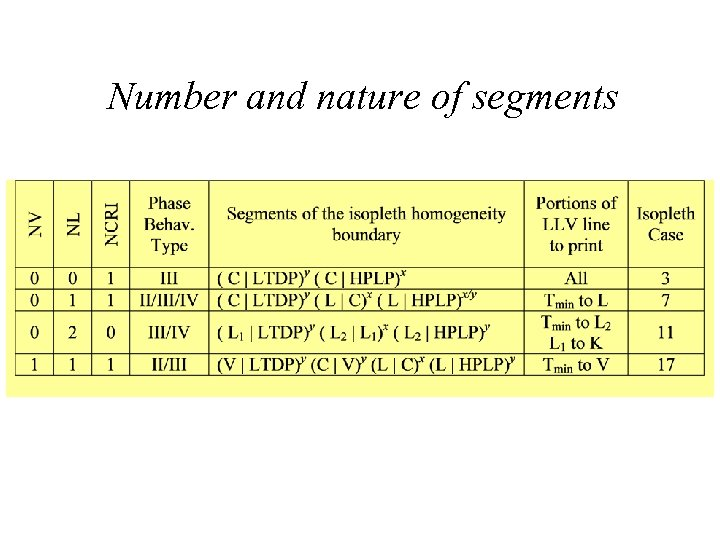Number and nature of segments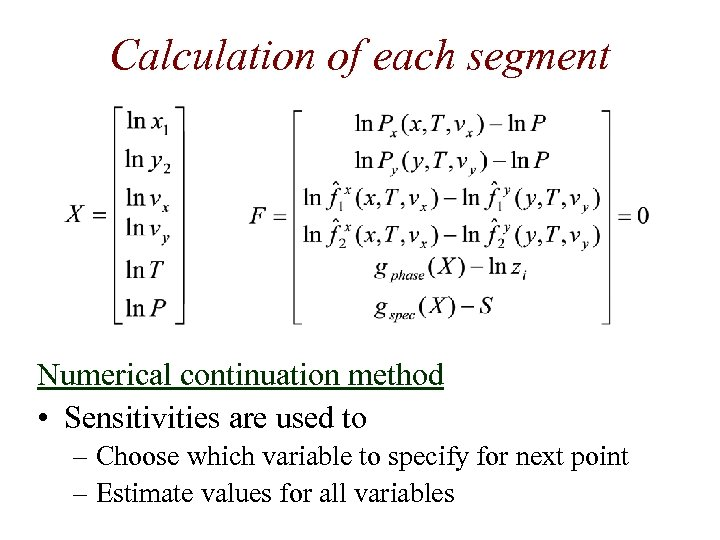Calculation of each segment Numerical continuation method • Sensitivities are used to – Choose which variable to specify for next point – Estimate values for all variables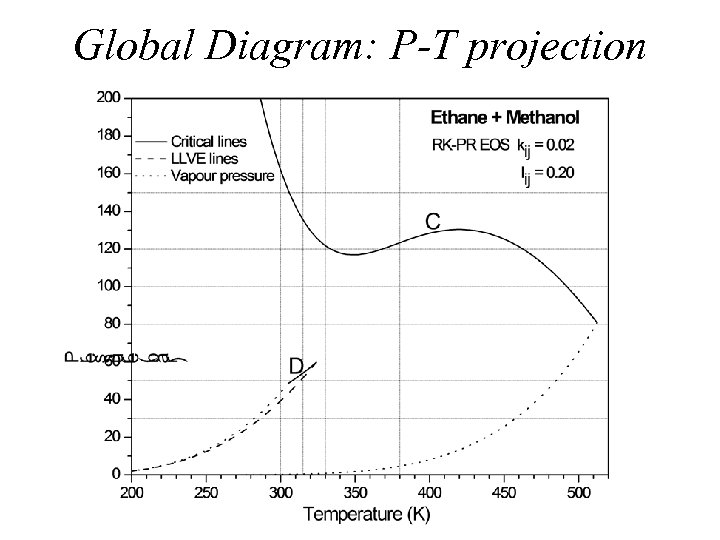Global Diagram: P-T projection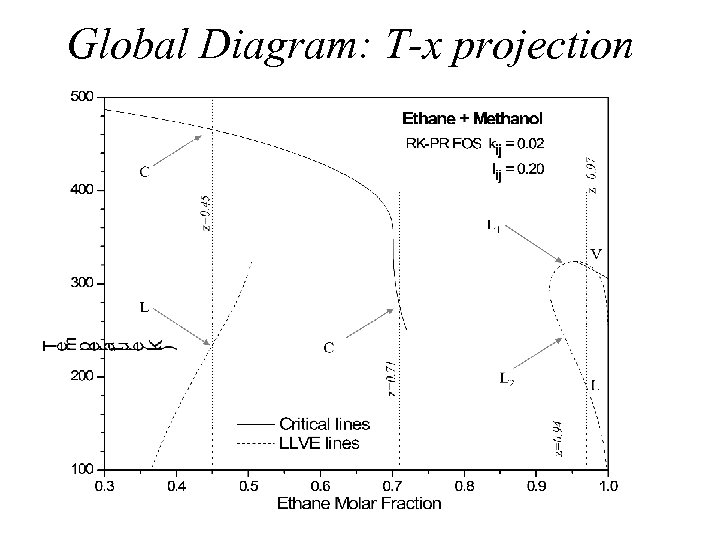Global Diagram: T-x projectionEthane-Methanol. RK-PR EOS.Ethane-Methanol. RK-PR EOS.Ethane-Methanol. RK-PR EOS.Ethane-Methanol. RK-PR EOS.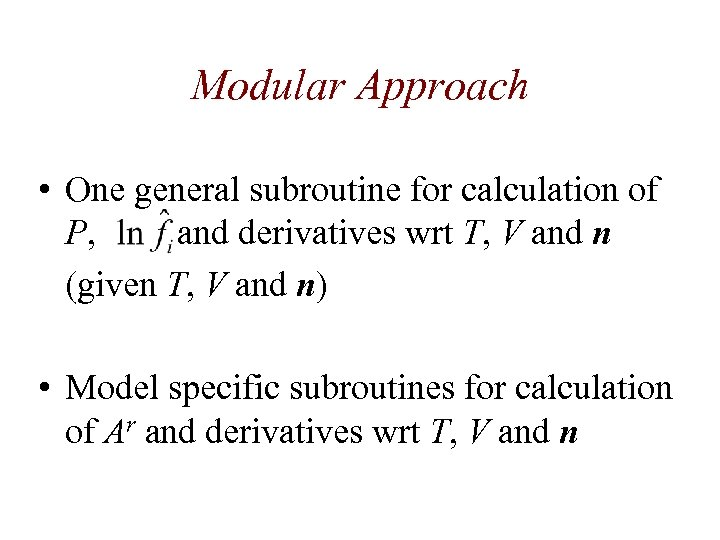Modular Approach • One general subroutine for calculation of P, and derivatives wrt T, V and n (given T, V and n) • Model specific subroutines for calculation of Ar and derivatives wrt T, V and n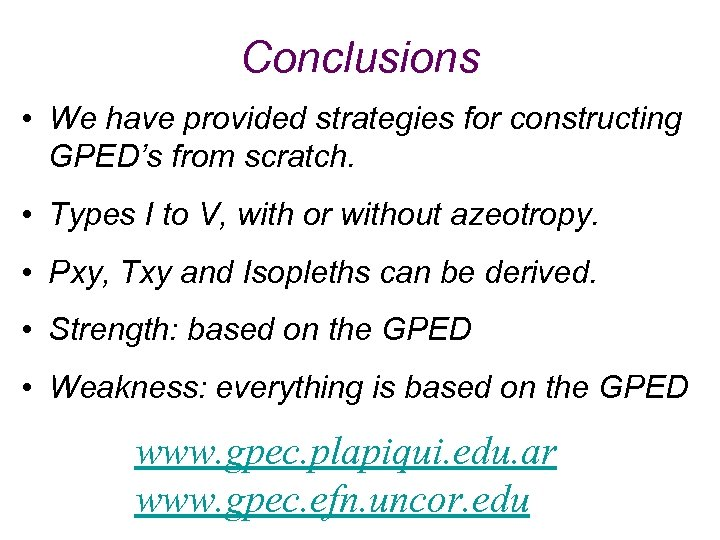Conclusions • We have provided strategies for constructing GPED’s from scratch. • Types I to V, with or without azeotropy. • Pxy, Txy and Isopleths can be derived. • Strength: based on the GPED • Weakness: everything is based on the GPED www. gpec. plapiqui. edu. ar www. gpec. efn. uncor. eduReferences • Global phase equilibrium calculations – – – • Cismondi, M. , Michelsen, M. “Global Phase Equilibrium Calculations: Critical Lines, Critical End Points and Liquid-Vapour Equilibrium in Binary Mixtures”. The Journal of Supercritical Fluids, Vol. 39, 287 -295. 2007. Cismondi, M. , Michelsen, M. “Automated Calculation of Complete Pxy and Txy Diagrams for Binary Systems”. Fluid Phase Equilibria, Vol. 259, 228 -234. 2007. Cismondi, M. , Michelsen, M. L. , Zabaloy, M. S. “Automated generation of phase diagrams for binary systems with azeotropic behavior”. Industrial and Engineering Chemistry Research, Vol. 47 Issue 23, 9728– 9743. 2008. GPEC (the program) – • Cismondi, M. , Nuñez, D. N. , Zabaloy, M. S. , Brignole, E. A. , Michelsen, M. L. , Mollerup, J. M. “GPEC: A Program for Global Phase Equilibrium Calculations in Binary Systems” (Oral Presentation). EQUIFASE 2006. Morelia, Michoacán, México. October 21 -25, 2006. Models and their pure compound parameters – – Cismondi, M. , Mollerup, J. “Development and Application of a Three-Parameter RK-PR Equation of State”. Fluid Phase Equilibria, Vol. 232, 74 -89. 2005. Cismondi, M. , Brignole, E. A. , Mollerup, J. “Rescaling of Three-Parameter Equations of State: PC-SAFT and SPHCT”. Fluid Phase Equilibria, Vol. 234, 108 -121. 2005.